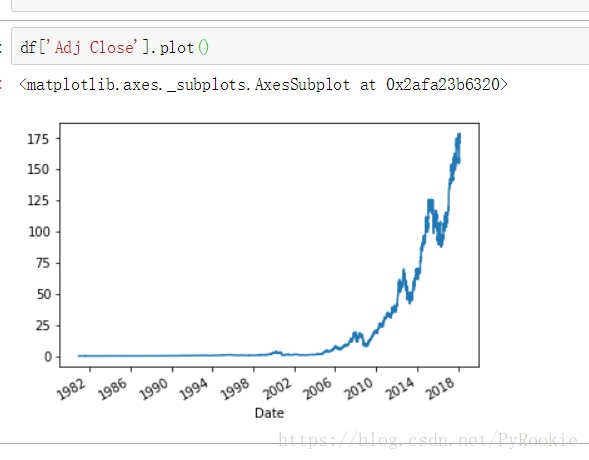# Python高級數據分析之pandas和matplotlib繪圖

## 一、matplotlib 庫

`import matplotlib.pyplot as plt`

```>>> jin = plt.imread("./jin.png")
>>> jin
array([[[0.24313726, 0.24313726, 0.24705882],
...,

[0.7294118 , 0.7294118 , 0.7294118 ]]], dtype=float32)

>>> jin.shpae
(273, 411, 3)
'''

'''
```

2）plt.imshow(ndarray)顯示圖片

```>>> plt.imshow(nd)  #將加載後的多維數組傳入就可以將圖片渲染出來
'''

'''
```

3）plt.imsave(ndarray)保存圖片

`plt.imsave(ndarray)`

```plt.imsave('圖片名稱.png',圖片數據,cmap='gray')    #保存圖片
cmap:將圖片保存為黑白圖片
```

## 二、Pandas繪圖

### 1.繪制簡單的線型圖

#### 1.1）簡單的Series圖表示例 .plot()

```# 正弦曲線
x = np.linspace(0,2*np.pi,100)      # 從0 到 2π  取100份
y = np.sin(x)                       # y 為sin(x) 的值
s = Series(data=y,index=x)          # 構建一個Series對象
s.plot()                  # 使用Series的plot()方法```#### 1.2) 兩個Series繪制的曲線可以疊加2）簡單的DataFrame圖表示例 .plot()

```x = np.linspace(0,2*np.pi,100)          # 從0 到 2π  取100份
df = DataFrame(data={'sin':np.sin(x),'cos':np.cos(x)},index=x)   #創建DataFrame對象
df.plot()```### 2.數據驅動的線型圖(分析蘋果股票)

```import numpy as np
import pandas as pd
from pandas import Series,DataFrame```

1.讀取數據

```df = pd.read_csv('./data/AAPL.csv')2.檢查數據類型

df.dtypes

Date          object       #時間的數據類型為對象,在使用中需要做處理
Open         float64
High         float64
Low          float64
Close        float64
Volume       float64
dtype: object

3.將’Date’這行數據轉換為時間數據類型

pd.to_datetime(Series對象)

```df['Date']  # 這一列獲取出來是一個Series
type(df['Date'])
df['Date'] = pd.to_datetime(df['Date'])```

df.dtypes

Date         datetime64[ns]  #此時已經是時間類型的數據瞭
Open                float64
High                float64
Low                 float64
Close               float64
Volume              float64
dtype: object

4.將’Date’設置為行索引

```df.set_index('Date',inplace=True)
'''
inplace:改變原來的變量的值
'''
```

`df['Adj Close'].plot()`### 3.繪制簡單的柱狀圖

1) Series柱狀圖示例,kind = ‘bar’/’barh’

```'''
data:  生成之後為Y軸的數據
index: 生成之後為X軸的索引
'''
s = Series(data=np.random.randint(0,10,size=5),index=list('abcde'))  #隨機產生5個數
s.plot(kind='bar')``````'''
data:  生成之後為Y軸的數據
index: 生成之後為X軸的索引
'''
s = Series(data=np.random.randint(0,10,size=5),index=list('abcde'))
s.plot(kind='barh')```2) DataFrame柱狀圖示例

```'''
index: 生成數據X軸的索引
columns: 特征的名稱
'''
df = DataFrame(np.random.randint(0,10,size=(8,4)),index=list('abcdefgh'),columns=list('ABCD'))
df.plot(kind='bar')```### 4.繪制簡單的直方圖

```s = Series(np.random.normal(loc=0,scale=5,size=10000))    # normal 正態分佈
s.hist()
#這裡模擬出一組以0為中心,標準差為5的正態分佈數據, 繪制出的直方圖如下```### 5.繪制簡單的核密度(“ked”)圖

```Series(np.random.normal(loc=0,scale=5,size=10000))
s.plot(kind='kde')``````ndarr1 = np.random.normal(loc=-10,scale=5,size=5000)  #第一組數據
ndarr2 = np.random.normal(loc=15,scale=2,size=5000)  第二組數據
ndarr = np.concatenate([ndarr1,ndarr2])   #將兩組數據合為一組
s = Series(ndarr)    #生成Series對象
s.plot(kind='kde')  # 核心 密度 估計 用來展示某個位置出現內容的概率的估計值
s.hist(density=True)  # density表示直方圖也以 密度概率的值來展示  hist用來展示某個位置出現內容的實際頻率
#展示效果如下```
```'''
s.plot(kind='kde')  展示某個位置的估計值
s.hist(density=True)  表示某個位置出現內容的實際頻率
'''```### 6.繪制簡單的散點圖

```df = DataFrame(np.random.randint(0,150,size=(20,3)),columns=['python','math','eng'])
df   #模擬一個DataFrame數據，列名為'python','math','eng'

python  math    eng
0   141     41      55
1   37      76      96
2   61      28      135
。。。
17  124     103     83
18  86      47      44
19  35      85      85```

```df.plot(kind='scatter',x='python',y='eng')
# kind = 'scatter'  ， 給明標簽columns``````np.random.randint(-10,10,1)   # 0-10之間 隨機取一個數
df['php'] = df['python'].map(lambda x: x*0.9+np.random.randint(-10,10,1))
df

python  math    eng php
0   121     67      15  113.9
1   148     33      149 123.2
。。。
18  79      77      108 74.1
19  105     107     53  102.5```